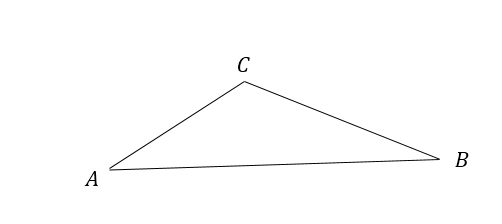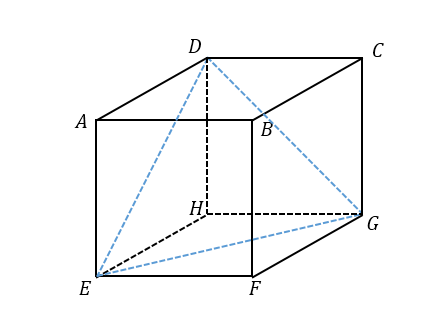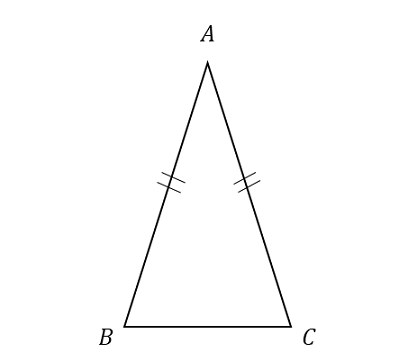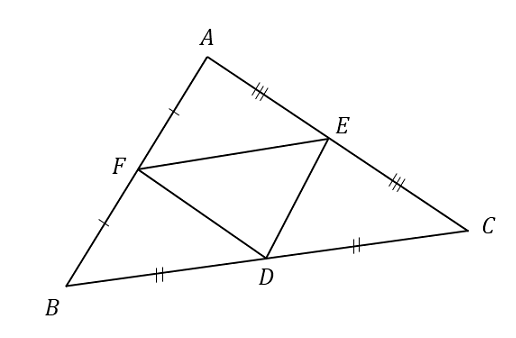Geometry

# Triangles - Classification By AnglesIf $\angle A = 45 ^\circ, \angle B = 35 ^\circ$ and $\angle C = 100 ^\circ$ in the above triangle, what type of triangle is $\triangle ABC?$If $ABCDEFGH$ is a cube with side length $5,$ what is $\angle DEG ?$The length of $\overline {AB}$ is the same as that of $\overline {AC}$ in the above triangle. If $\angle B =74^\circ,$ what is $\angle C ?$Suppose that $\angle A = 80 ^\circ, \angle B = 55 ^\circ$ and $\angle C = 45 ^\circ .$ If points $D, E$ and $F$ are the midpoints of $\overline{BC}, \overline{CA}$ and $\overline{AB},$ respectively, what type of triangle is $\triangle DEF ?$

Note: The above diagram is not drawn to scale.

What do we call a triangle which has exactly 2 equal angles?

×